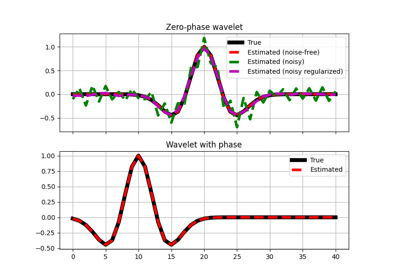# pylops.avo.prestack.PrestackWaveletModelling¶

pylops.avo.prestack.PrestackWaveletModelling(m, theta, nwav, wavc=None, vsvp=0.5, linearization='akirich')[source]

Pre-stack linearized seismic modelling operator for wavelet.

Create operator to be applied to a wavelet for generation of band-limited seismic angle gathers using a linearized version of the Zoeppritz equation.

Parameters: m : np.ndarray elastic parameter profles of size $$[n_{t_0} \times N]$$ where $$N=3,\,2$$. Note that the dtype of this variable will define that of the operator theta : int Incident angles in degrees. Must have same dtype of m (or it will be automatically cast to it) nwav : np.ndarray Number of samples of wavelet to be applied/estimated wavc : int, optional Index of the center of the wavelet vsvp : np.ndarray or float, optional $$V_S/V_P$$ ratio linearization : {“akirich”, “fatti”, “PS”} or callable, optional “akirich”: Aki-Richards. See pylops.avo.avo.akirichards. “fatti”: Fatti. See pylops.avo.avo.fatti. “PS”: PS. See pylops.avo.avo.ps. Function with the same signature as pylops.avo.avo.akirichards Mconv : LinearOperator pre-stack modelling operator for wavelet estimation. NotImplementedError If linearization is not an implemented linearization

Notes

Pre-stack seismic modelling for wavelet estimate is the process of constructing seismic reflectivities using three (or two) profiles of elastic parameters in time (or depth) domain arranged in an input vector $$\mathbf{m}$$ of size $$n_{t_0} \times N$$:

$d(t, \theta) = \sum_{i=1}^N G_i(t, \theta) m_i(t) * w(t)$

where $$w(t)$$ is the time domain seismic wavelet. In compact form:

$\mathbf{d}= \mathbf{G} \mathbf{w}$

On the other hand, pre-stack wavelet estimation aims at recovering the wavelet given knowledge of the band-limited seismic pre-stack data and the elastic parameter profiles.

## Examples using pylops.avo.prestack.PrestackWaveletModelling¶Wavelet estimation